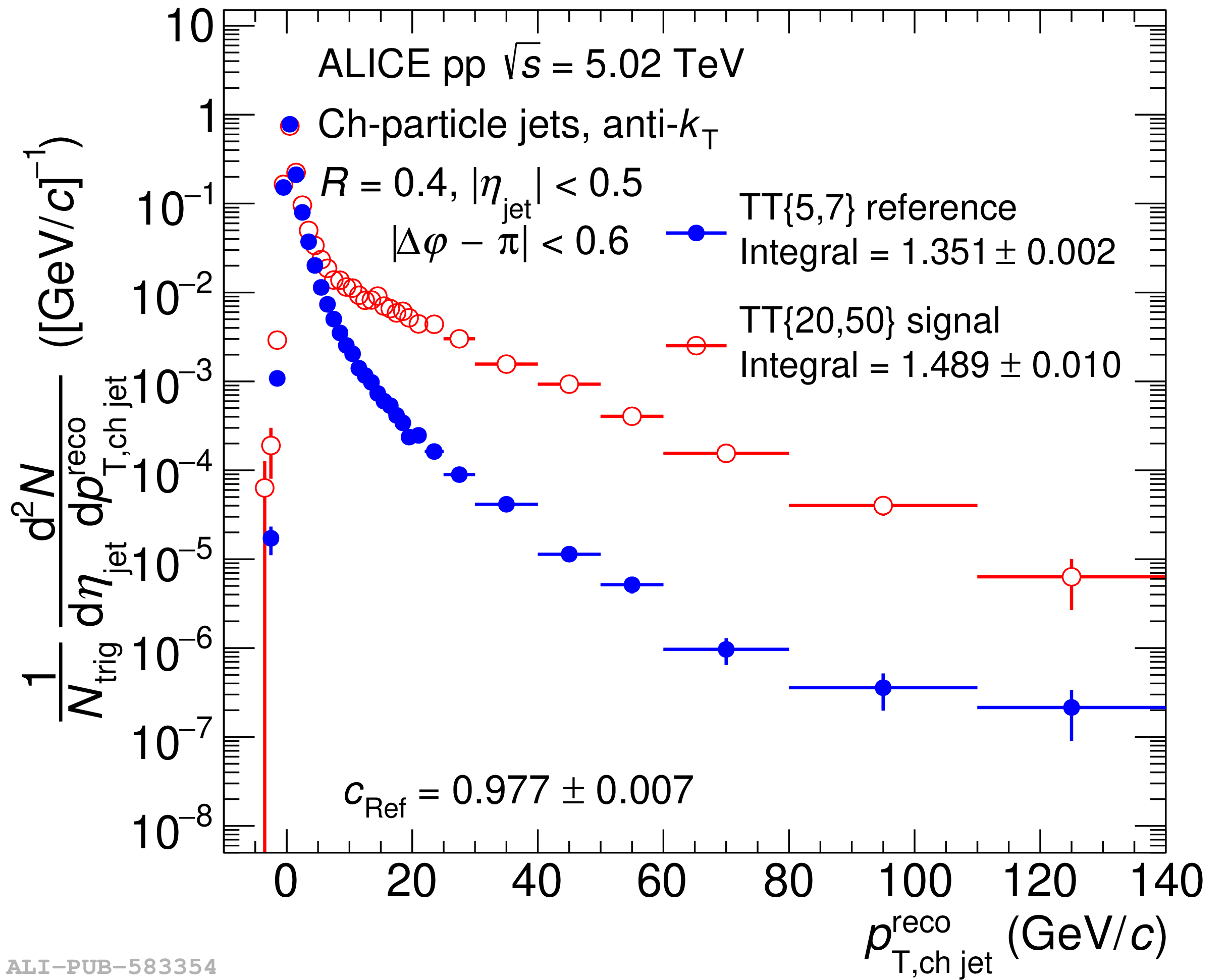# Figure 7

 Left: trigger-normalized semi-inclusive recoil jet distributions with $\rr=0.4$ for \TTSig\ and \TTRef-selected populations in \pp\ collisions at $\s=5.02$ \TeV; Right: ratio of the two distributions. The horizontal blue line indicates the fit to the ratio close to $\pTreco=0$ for the determination of \cRef, the value of which is also given in the figures.